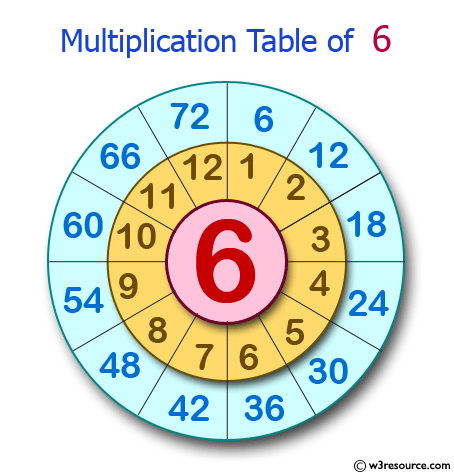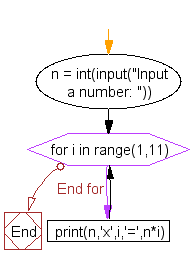﻿ Python Exercise: Create the multiplication table of a number - w3resource# Python Exercise: Create the multiplication table of a number

## Python Conditional: Exercise - 43 with Solution

Write a Python program to create the multiplication table (from 1 to 10) of a number.

Pictorial Presentation:Sample Solution:

Python Code:

``````n = int(input("Input a number: "))

# use for loop to iterate 10 times
for i in range(1,11):
print(n,'x',i,'=',n*i)
```
```

Sample Output:

```Input a number: 5
5 x 1 = 5
5 x 2 = 10
5 x 3 = 15
5 x 4 = 20
5 x 5 = 25
5 x 6 = 30
5 x 7 = 35
5 x 8 = 40
5 x 9 = 45
5 x 10 = 50
```

Flowchart :## Visualize Python code execution:

The following tool visualize what the computer is doing step-by-step as it executes the said program:

Python Code Editor:

Have another way to solve this solution? Contribute your code (and comments) through Disqus.

What is the difficulty level of this exercise?

Test your Python skills with w3resource's quiz

﻿

## Python: Tips of the Day

Returns a list with n elements removed from the beginning

Example:

```def tips_take(itr, n = 1):
return itr[:n]
print(tips_take([1, 2, 3], 5))
print(tips_take([1, 2, 3], 0))
```

Output:

```[1, 2, 3]
[]
```